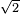# 3.1.3: Mean Free Path

$$\newcommand{\vecs}{\overset { \rightharpoonup} {\mathbf{#1}} }$$ $$\newcommand{\vecd}{\overset{-\!-\!\rightharpoonup}{\vphantom{a}\smash {#1}}}$$$$\newcommand{\id}{\mathrm{id}}$$ $$\newcommand{\Span}{\mathrm{span}}$$ $$\newcommand{\kernel}{\mathrm{null}\,}$$ $$\newcommand{\range}{\mathrm{range}\,}$$ $$\newcommand{\RealPart}{\mathrm{Re}}$$ $$\newcommand{\ImaginaryPart}{\mathrm{Im}}$$ $$\newcommand{\Argument}{\mathrm{Arg}}$$ $$\newcommand{\norm}{\| #1 \|}$$ $$\newcommand{\inner}{\langle #1, #2 \rangle}$$ $$\newcommand{\Span}{\mathrm{span}}$$ $$\newcommand{\id}{\mathrm{id}}$$ $$\newcommand{\Span}{\mathrm{span}}$$ $$\newcommand{\kernel}{\mathrm{null}\,}$$ $$\newcommand{\range}{\mathrm{range}\,}$$ $$\newcommand{\RealPart}{\mathrm{Re}}$$ $$\newcommand{\ImaginaryPart}{\mathrm{Im}}$$ $$\newcommand{\Argument}{\mathrm{Arg}}$$ $$\newcommand{\norm}{\| #1 \|}$$ $$\newcommand{\inner}{\langle #1, #2 \rangle}$$ $$\newcommand{\Span}{\mathrm{span}}$$$$\newcommand{\AA}{\unicode[.8,0]{x212B}}$$

The mean free path is the average distance traveled by a moving molecule between collisions.

## Introduction

Imagine gas leaking out of a pipe. It would take a while for the gas to diffuse and spread into the environment. This is because gas molecules collide with each other, causing them to change in speed and direction. Therefore, they can never move in a straight path without interruptions. Between every two consecutive collisions, a gas molecule travels a straight path. The average distance of all the paths of a molecule is the mean free path.

## Analogy

Imagine a ball traveling in a box ; the ball represents a moving molecule. Every time the ball hits a wall, a collision occurs and the direction of the ball changes (Figure 1). The ball hits the wall five times, causing five collisions. Between every two consecutive collisions, the ball travels an individual path. It travels a total of four paths between the five collisions; each path has a specific distance, d. The mean free path, \lambda, of this ball is the average length of all four paths.Figure 1. This figure shows a ball traveling in a box.

Each path traveled by the ball has a distance, denoted dn:

$\lambda = \dfrac{d_1 + d_2 + d_3 + d_4}{4} \nonumber$

### Calculations

In reality, the mean free path cannot be calculated by taking the average of all the paths because it is impossible to know the distance of each path traveled by a molecule. However, we can calculate it from the average speed ($$\langle c \rangle$$) of the molecule divided by the collision frequency ($$Z$$). The formula for this is:

$\lambda = \dfrac{\langle c \rangle}{Z} \nonumber$

Because $$Z$$ is equal to $$1/ t$$, where $$t$$ is the average time between collisions, the formula can also be:

$\begin{eqnarray} \lambda &=& \dfrac{\langle c \rangle}{\dfrac{1}{t}} \\ &=& \langle c \rangle \times t \end{eqnarray} \nonumber$

$\lambda = \sqrt{2} \pi d^2 \langle c \rangle (N/V) \nonumber$

where

• $$d$$ is the diameter of the molecule and
• ($$N/V$$) is the density,

The formula can be further modified to:

\begin{align*} \lambda &= \dfrac{\langle c \rangle}{\sqrt{2} \pi d^2 \langle c \rangle \dfrac{N}{V}} \\[4pt] &= \dfrac{1}{\sqrt{2} \pi d^2 \dfrac{N}{V}} \end{align*}

### Factors affecting mean free path

• Density: As gas density increases, the molecules become closer to each other. Therefore, they are more likely to run into each other, so the mean free path decreases.
• Increasing the number of molecules or decreasing the volume causes density to increase. This decreases the mean free path.
• Radius of molecule: Increasing the radii of the molecules decreases the space between them, causing them to run into each other more often. Therefore, the mean free path decreases.
• Pressure, temperature, and other factors that affect density can indirectly affect mean free path.
##### Exercise $$\PageIndex{1}$$

A gas has an average speed of 10 m/s and a collision frequency of 10 s-1. What is its mean free path?

l = <c> / = 10 m/s / 10 s^(-1) = 1 m

##### Exercise $$\PageIndex{2}$$

A gas has an average speed of 10 m/s and an average time of 0.1 s between collisions. What is its mean free path?

l = <c> X (average time between collisions) = 10 m/s X 0.1 s = 1 m

##### Exercise $$\PageIndex{3}$$
1. A gas has an average speed of 10 m/s and an average time of 0.1 s between collisions. What is its mean free path?
2. A gas has a density of 10 particles m-3 and a molecular diameter of 0.1 m. What is its mean free path?

l = 1 / [$\sqrt{2}$ (pi) d^2 (N/v)] = 1 / [$\sqrt{2}$ (pi)(0.1 m)^2 (10 m^(-3))] = 2.25 m

##### Exercise $$\PageIndex{4}$$

A gas in a 1 m3 container has a molecular diameter of 0.1 m. There are 10 molecules. What is its mean free path?

l = 1 / [$\sqrt{2}$ (pi) d^2 (N/v)] = 1 / [$\sqrt{2}$ (pi)(0.1 m)^2 (10 / 1 m^3)] = 2.25 m

##### Exercise $$\PageIndex{5}$$

A gas has a molecular diameter of 0.1 m. It also has a mean free path of 2.25 m. What is its density?

l = 1 / [$\sqrt{2}$ (pi) d^2 (N/v)]
2.25 m = 1 / [$\sqrt{2}$ (pi)(0.1 m)^2 (N/v)]